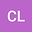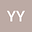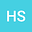An upwind multistep difference-mixed volume element method for a positive semi-definite contamination treatment from nuclear waste
•••• Changfeng Li,
• Yirang Yuan,
• Huailing Song
Changfeng Li
Shandong University

Corresponding Author:[email protected]

Author ProfileYirang Yuan
Shandong University
Author ProfileHuailing Song
Hunan University
Author Profile## Abstract

A three-dimensional contamination treatment problem from nuclear waste in porous media, where the diffusion matrix is generally positive semi-definite, is discussed in this paper. The mathematical model is defined by a nonlinear initial-boundary system consisting of partial different equations. An elliptic equation, two convection-diffusion equations and a heat conductor equation are given to determine the pressure, the concentrations of brine and radionuclide, and the temperature, respectively. The concentration equations and heat conductor equation include Darcy velocity dependent on the pressure, and their physical motions are affected. A conservative mixed volume element is used to approximate the pressure, and one-order computational accuracy is improved for Darcy velocity. The concentrations of brine and radionuclide and the temperature are solved by an upwind multiste difference-mixed volume element, where the derivative to time is approximated by a multistep difference, the diffusions and convection terms are treated by a mixed volume element and an upwind approximation, respectively. This composite method can eliminate numerical dispersion and nonphysical oscillations, so it can solve convection-dominated diffusion equations accurately and stably. The mixed volume element can obtain the concentrations, temperature and their adjoint vectors simultaneously, and it has the conservation of mass or energy. This physical nature is important in numerical simulation of underground fluid flow problems. Applying the theory and special treatment of a priori estimates, we obtain optimal order estimates in L2-norm. Numerical experiments show the efficiency and applicability in simulating contamination treatment from nuclear waste.